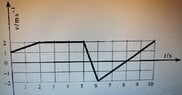# Why is the result for distance traveled different using different approaches?

Callmelucky
Homework Statement:
calculate the distance traveled by a body given the graph
Relevant Equations:
##d=V_0\times t + \frac{1}{2}\times at^{2}##, ##d=\frac{vt}{2}##
So basically I wonder why the distance traveled by a body in the 5th second gives different results when calculated by the formula for accelerating body(##d=V_0\times t + \frac{1}{2}\times at^{2}##) and when calculated using a graph(formula for the surface of the triangle).

Here is the graph of the problem(pic below).
When I calculate distance traveled using ##d=\frac{vt}{2}## I get ##d=\frac{\Delta v\Delta t}{2}=\frac{4\times 1}{2}=2m##, and when I do the same using ##d=V_0\times t + \frac{1}{2}\times at^{2}## I get ##d=V_0\times t+\frac{1}{2}\times at^{2} = 2 + (-2)=0## because ##a=\frac{\Delta v}{\Delta t}= -4##.

If someone can please tell me where I am wrong, thank you.

#### Attachments

•WhatsApp Image 2023-03-17 at 19.04.43.jpeg
47.2 KB · Views: 5

Homework Helper
Where does the d=vt/2 expression come from ?

Gold Member
MHB
my textbook, it's just marked as s instead of d
There must be a typo. The area of the shaded portion (a trapezoid) is
##d = \dfrac{(v_0+v)t}{2} ##

and this formula will give consistent results with the other.

-Dan

•Callmelucky
Callmelucky
There must be a typo. The area of the shaded portion (a trapezoid) is
##d = \dfrac{(v_0+v)t}{2} ##

and this formula will give consistent results with the other.

-Dan
Does that then mean that the body didn't cross any distance for that one second?
One more question, I have calculated that the average speed in the first 6 seconds is 3/2 m/s but the solution in the textbook is 10/6 m/s. I used the same formula for distance traveled by accelerating the body for the first 2 seconds which is ##d=V_0\times t + \frac{1}{2}\times at^{2} = 1\times 2+\frac{1}{2}\times 0.5\times 4= 3m##, after that, distance traveled from 2nd to 5th second is 6m(v*t=2*3=6m) and distance from 5th to 6th second is 0m. So that is 9m/6sec= 3/2 m/s but the solution in the textbook is 10/9 m/s, can you please explain how?
Thank you

Homework Helper
Gold Member
One more question, I have calculated that the average speed in the first 6 seconds is 3/2 m/s but the solution in the textbook is 10/6 m/s. I used the same formula for distance traveled by accelerating the body for the first 2 seconds which is ##d=V_0\times t + \frac{1}{2}\times at^{2} = 1\times 2+\frac{1}{2}\times 0.5\times 4= 3m##, after that, distance traveled from 2nd to 5th second is 6m(v*t=2*3=6m) and distance from 5th to 6th second is 0m. So that is 9m/6sec= 3/2 m/s but the solution in the textbook is 10/9 m/s, can you please explain how?
Thank you
The distance traveled from from the 5th to the 6th second is not zero. The displacement is zero. The distance traveled is the sum of the "go" trip (5.0-5.5 s) and the "return" trip (5.5-6.0 s.) Average speed is what the odometer records over the entire trip divided by the duration of the trip.

•topsquark
•